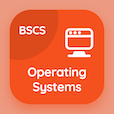Class 10 Online Courses

Grade 10 Math MCQ PDF - Chapters

# Sets and Functions Multiple Choice Questions Online p. 1

Learn Sets and functions multiple choice questions and answers, sets and functions quiz answers PDF to learn grade 10 math test 1 for online courses. Recognize of Operations on Sets MCQs, sets and functions quiz questions and answers for online schools. "Sets and Functions MCQ" PDF Book: recognize of operations on sets, binary relation, sets test prep for online high school courses.

"If 2 sets A and B are given, then the set consisting of all the elements which are either in A or in B or in both is called" Multiple Choice Questions (MCQ) on sets and functions with choices union of a and b, intersection of a and b, complement of a, and complement of b for online schools. Practice recognize of operations on sets quiz questions for school certificate programs for online school and college.

## MCQs on Sets and Functions Quiz

MCQ: If 2 sets A and B are given, then the set consisting of all the elements which are either in A or in B or in both is called

Intersection of A and B
union of A and B
Complement of A
Complement of B

MCQ: The set consisting of all the first elements of each ordered pair in the relation is called

subset
domain of relation
range of relation
complement of a set

MCQ: If U = {1,2,3,4,5} and A = {2,4} then A' should be

{2,4,5}
{2,4}
{1,2,3,4,5}
{1,3,5}

MCQ: The number of elements in power set {1, 2, 3} are

5
6
7
8

MCQ: The range of R = {(0, 2), (2, 4), (3, 4), (4, 5)} is

{0, 2, 4, 5}
{0, 2, 3, 4}
{2, 4, 5}
{3, 4, 5}